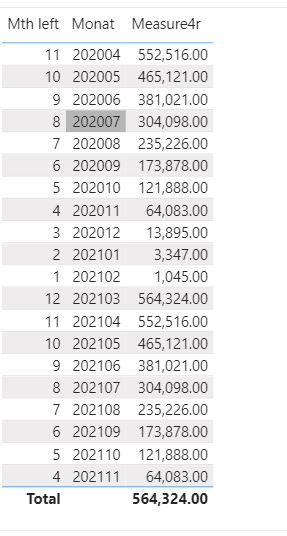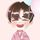cancel
Showing results for
Did you mean:Helper I

## Sum the remaining months

Hi,

I have the below table where I would like to have a measure that is sum the remaining month number.

The FY is starting at 2020.04 and end at 2021.03

The MTH left shows the remains number of month from the fiscal year.

For example we are now in December 202012(Monat) and I want a measure that sum the remaining mth number in this case this month and the remaining months (Mth left 3+2+1+0) = 6

January would be = 34 REPLIES 4Helper IHelper I

Thank your for your help, I tried the above measures and it seems like it's sum up every single rows.Helper I

I have 81744 rows and the column mth left 12 distinct valuesCommunity Support

Hi @Austinehype ,

You could use the following formula:

``````Measure =
CALCULATE (
SUM ( 'Table'[Mth left] ),
FILTER ( ALL ( 'Table' ), 'Table'[Mth left] <= MAX ( 'Table'[Mth left] ) )
)``````
``````Column =
CALCULATE (
SUM ( 'Table'[Mth left] ),
FILTER ( ALL ( 'Table' ), 'Table'[Date] >= EARLIER ( 'Table'[Date] ) )
)``````

Or you could add a Index column firstly and then use:

``````Measure 2 =
CALCULATE (
SUM ( 'Table'[Mth left] ),
FILTER ( ALL ( 'Table' ), 'Table'[Index] >= MAX ( 'Table'[Index] ) )
)``````

My visualization looks like this:Here is the pbix file.

If this post helps, then please consider Accept it as the solution to help the other members find it more quickly.

Best Regards,
Eyelyn Qin